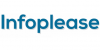• (a.) Tetragonal. • (a.) Pertaining to terms of the second degree; as, a quadratic equation, in which the highest power of the unknown quantity is a square. • (a.) Of or pertaining to a square, or to squares; resembling a quadrate, or square; square.adjective of or relating to the second power; `quadratic equation`
Found on https://www.encyclo.co.uk/local/20974[collection] Quadratic is a collection of four science fiction works by Olaf Stapledon and Murray Leinster. It was edited by William L. Crawford and published in 1953 by Fantasy Publishing Company, Inc. in an edition of 300 copies. The book is an omnibus of Stapledon`s Worlds of Wonder and Leinster`s Murder Madness, created by combining unb...Quad·rat'ic adjective [ Confer French quadratique .] 1. Of or pertaining to a square, or to squares; resembling a quadrate, or square; square. 2. (Crystallog.) Tetragonal. 3. (Alg.) Pertaining to terms of the second degree; as, a quadratic equation...
Found on http://www.encyclo.co.uk/webster/Q/1An expression or an equation that contains the variable squared, but not raised to any higher power. For instance a quadratic equation in x contains x2 but not x3. Similarly a quadratic expression, or a quadratic form, contains its variable(s) squared bu...quadratic, mathematical expression of the second degree in one or more unknowns (see polynomial). The general quadratic in one unknown has the form ax2+bx+c, where a, b, and c are constants and x is the variable. A quadratic equation ax2+bx+c=0 always has two roots, not necessarily distinct; these m...[adj] - of or relating to or resembling a square 2. [adj] - of or relating to the second power 3. [n] - a polynomial of the second degree